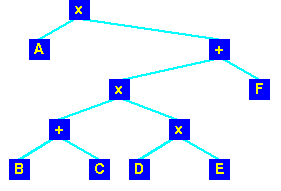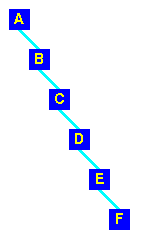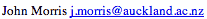Data Structures and Algorithms 8 Searching Revisited

Before we examine some more searching techniques, we need to consider some operations on trees - in particular means of traversing trees.

Tree operations

A binary tree can be traversed in a number of ways:
 pre-order Visit the root Traverse the left sub-tree, Traverse the right sub-tree in-order Traverse the left sub-tree, Visit the root Traverse the right sub-tree post-order Traverse the left sub-tree, Traverse the right sub-tree Visit the root

If we traverse the standard ordered binary tree in-order, then we will visit all the nodes in sorted order.

Parse trees

 If we represent the expression: A*(((B+C)*(D*E))+F) as a tree:then traversing it post-order will produce:
A B C + D E * * F + *
which is the familiar reverse-polish notation used by a compiler for evaluating the expression.

Search Trees

We've seen how to use a heap to maintain a balanced tree for a priority queue. What about a tree used to store information for retrieval (but not removal)? We want to be able to find any item quickly in such a tree based on the value of its key. The search routine on a binary tree:
tree_search(tree T, Key key) {
if (T == NULL) return NULL;
if (key == T->root) return T->root;
else
if (key < T->root) return tree_search( T->left, key );
else               return tree_search( T->right, key );
}
is simple and provides us with a O(log n) searching routine as long as we can keep the tree balanced. However, if we simply add items to a tree, producing an unbalanced tree is easy!
 This is what happens if we add the letters A B C D E F in that order to a tree: Not exactly well balanced!Key terms

Pre-order tree traversal
Traversing a tree in the order: root | left | right
In-order tree traversal
Traversing a tree in the order: left | root | right
Post-order tree traversal
Traversing a tree in the order: left | right | root

©, 1998